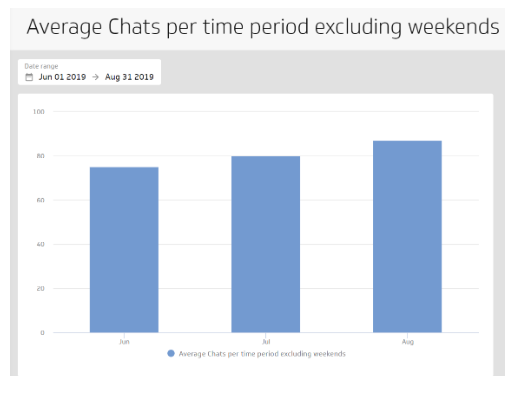I want to count the number of customer chats my team handles and compare this data over various time periods. My data has 1 row for each event and the following PowerMetric is based on a text field so my aggregation choices are Count or Count unique.  At a glance, it may appear that the chart shows the week of September 16 has lighter volume than the previous weeks, but that is because the week of September 16 is not yet finished.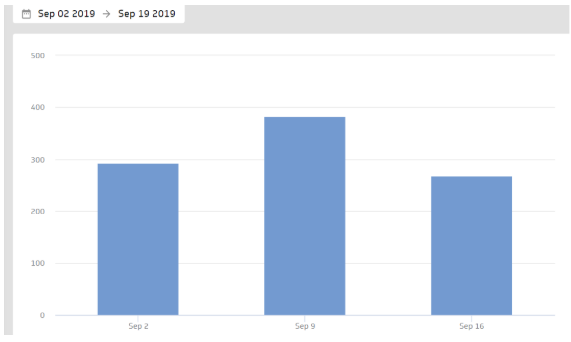To normalize this data and more easily determine the trend, I want to report on the average chats per day over various time periods, such as weekly or monthly. To create a PowerMetric that shows the average number of chats per day, I edit the modelled data source to group and count the number of chats per day using the GROUP and COUNTDISTINCT functions.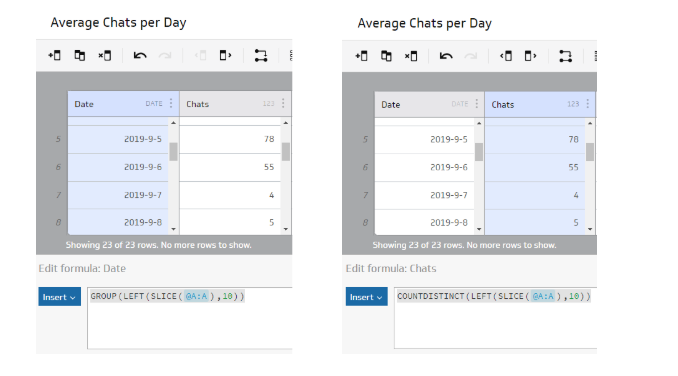Then, to build a PowerMetric using the above model:

1. Set Measure to Chats.
2. Set Default aggregation to Average
3. Select your type of data: Periodic summary by Day.

This PowerMetric shows me that our volume is up in the week of September 16.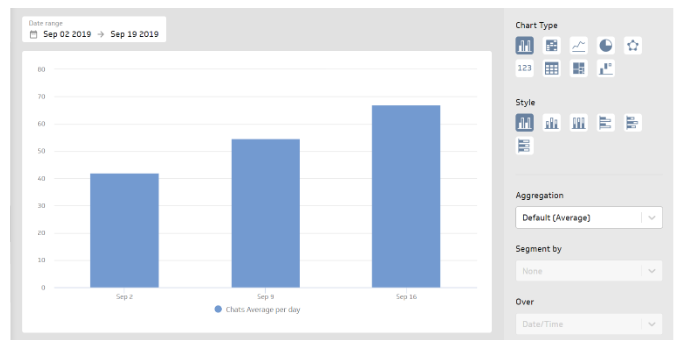This approach can be applied to any transactional data where records (rows) are counted.

How about if I want to exclude weekend days when calculating the average? If my data does not include weekends, the above PowerMetric will work, but if it contains weekend dates, I can add another column, Day of Week Count, to my modelled data source to indicate whether or not it is a day I want to include.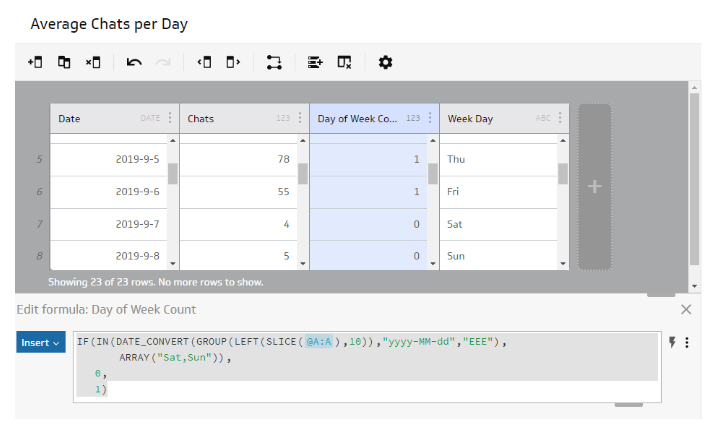Using Day of Week Count as the measure (Default aggregation is Sum, Type of data is Transactional values), I create a simple PowerMetric that I will then use to create a calculated PowerMetric.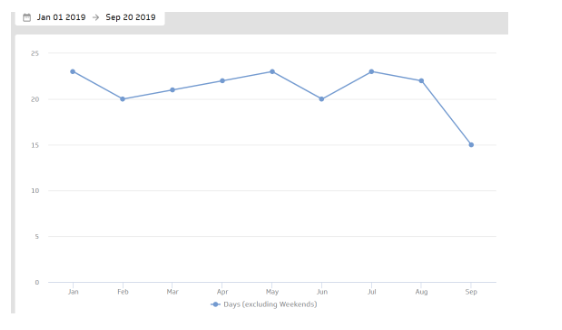This PowerMetric tells me there are 22 week days in August 2019, for example. Together with my original PowerMetric, I can divide the number of chats for a given period by the number of weekdays in that period, resulting in the average number of chats per week day. The formula for the calculated PowerMetric looks like:Note in this case Aggregation is set to Sum for both PowerMetrics because, to calculate the average over a time period, I need to divide the sum of the denominator by the sum the numerator.

The resulting PowerMetric shows me the average chats per day (excluding weekends)  for June, July and August.